# Complex Results

Results in some analysis types come as complex numbers.

A complex number z consists of a real and imaginary part, and can be written (in Cartesian format) as: z = x + iy
Where:
• x is the real part of z.
• y is the imaginary part of z.

Complex number can also be written in the polar format:

Where:
• x is the real part of z.
• y is the imaginary part of z.

Complex number can also be written in the polar format: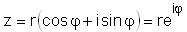where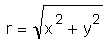, is the magnitude of z.

and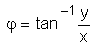, is the phase of z.

A response of a point under sinusoidal excitation (oscillation) can be described as: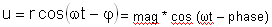Where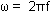, is the angular velocity and t is time. In HyperLife, you can select the increment of ωt (called "angle") from the Animation Controls panel.

## Complex Result in HyperLife

When a complex result is loaded, HyperLife will automatically switch to modal animation mode.

In modal animation mode, there is selection to choose a complex filter.

The available complex filters are:
mag * cos (ωt- phase)
the response with varying angle or ωt (in degree).
mag
magnitude (r) of the complex result
phase
phase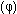of the complex number
real
real part (x) of the complex number
imaginary
imaginary part (y) of the complex number

Other functionalities can be viewed in Contour panel.

## Invariants for a Complex Vector or Tensor

Complex results of invariants of a vector (like magnitude of displacement) or a tensor (like von-Mises value of stress) can only be calculated at a specific angle from the response of each components at that angle. For example, complex responses of x, y and z components of displacement are: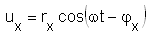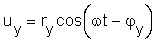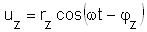For a specific angle ωt, the magnitude of displacement is calculated as: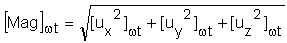Note that the response of an invariant is not a complex number. It can only be calculated at a specific angle. When an invariant is selected for modal animation, HyperLife will calculate the value at each specified angle increment for each response point separately.

## Plotting Complex Curves

You can view the curve plot from the Measure panel.

## View the Contour of a Complex Response at a Certain Angle

1. On the Animation toolbar, click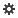.
2. From the Animation Controls panel, input the desired angle into the Current angle text field.
3. Press the Enter key on the keyboard.

## View the Contour Animation of a Complex Response

1. On the Animation toolbar, click.
2. From the Animation Controls panel, input the desired angle increment into the Increment by text field.
3. Click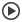.